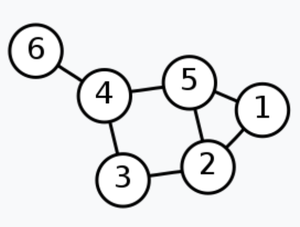Related Articles

# Java Program to Find Laplacian Matrix of an Undirected Graph

• Difficulty Level : Hard
• Last Updated : 17 Dec, 2020

The Laplacian matrix, also called the graph Laplacian, admittance matrix, Kirchhoff matrix or discrete Laplacian, is a matrix representation of a graph. To find the Laplacian matrix first, find adjacency matrix and degree matrix of a graph as the formula for the Laplacian matrix is as follows:

Laplacian matrix = Degree matrix – Adjacency matrix

Example```Laplacian Matrix:
2  -1   0   0  -1   0
-1   3  -1   0  -1   0
0  -1   2  -1   0   0
0   0  -1   3  -1  -1
-1  -1   0  -1   3   0
0   0   0  -1   0   1```

Basic definitions:

1. Adjacency matrix: Value can be either 0 or 1 according to graph vertices are connected to each other.
2. Degree matrix: Number of vertices adjacent to a vertex.

Algorithm:

1. Add appropriate edges of an undirected graph.
3. Compute the Laplacian matrix with the formula.

Below is the implementation of the above approach:

## Java

 `// Java program to find laplacian``// matrix of an undirected graph``class` `Graph {``    ``class` `Edge {``        ``int` `src, dest;``    ``}``    ``int` `vertices, edges;``    ``Edge[] edge;`` ` `    ``Graph(``int` `vertices, ``int` `edges)``    ``{``        ``this``.vertices = vertices;``        ``this``.edges = edges;``        ``edge = ``new` `Edge[edges];``        ``for` `(``int` `i = ``0``; i < edges; i++) {``            ``edge[i] = ``new` `Edge();``        ``}``    ``}``    ``public` `static` `void` `main(String[] args)``    ``{``        ``int` `i, j;``        ``int` `numberOfVertices = ``6``;``        ``int` `numberOfEdges = ``7``;``        ``int``[][] adjacency_matrix``            ``= ``new` `int``[numberOfEdges][numberOfEdges];``        ``int``[][] degree_matrix``            ``= ``new` `int``[numberOfEdges][numberOfEdges];``        ``int``[][] laplacian_matrix``            ``= ``new` `int``[numberOfEdges][numberOfEdges];``        ``Graph g``            ``= ``new` `Graph(numberOfVertices, numberOfEdges);`` ` `        ``// Adding edges with source and destination``        ``// edge 1--2``        ``g.edge[``0``].src = ``1``;``        ``g.edge[``0``].dest = ``2``;`` ` `        ``// edge 1--5``        ``g.edge[``1``].src = ``1``;``        ``g.edge[``1``].dest = ``5``;`` ` `        ``// edge 2--3``        ``g.edge[``2``].src = ``2``;``        ``g.edge[``2``].dest = ``3``;`` ` `        ``// edge 2--5``        ``g.edge[``3``].src = ``2``;``        ``g.edge[``3``].dest = ``5``;`` ` `        ``// edge 3--4``        ``g.edge[``4``].src = ``3``;``        ``g.edge[``4``].dest = ``4``;`` ` `        ``// edge 4--6``        ``g.edge[``5``].src = ``4``;``        ``g.edge[``5``].dest = ``6``;`` ` `        ``// edge 5--4``        ``g.edge[``6``].src = ``5``;``        ``g.edge[``6``].dest = ``4``;`` ` `        ``// Adjacency Matrix``        ``for` `(i = ``0``; i < numberOfEdges; i++) {``            ``for` `(j = ``0``; j < numberOfEdges; j++) {``                ``adjacency_matrix[g.edge[i].src]``                                ``[g.edge[i].dest]``                    ``= ``1``;``                ``adjacency_matrix[g.edge[i].dest]``                                ``[g.edge[i].src]``                    ``= ``1``;``            ``}``        ``}``        ``System.out.println(``"Adjacency matrix : "``);``        ``for` `(i = ``1``; i < adjacency_matrix.length; i++) {``            ``for` `(j = ``1``; j < adjacency_matrix[i].length;``                 ``j++) {``                ``System.out.print(adjacency_matrix[i][j]``                                 ``+ ``" "``);``            ``}``            ``System.out.println();``        ``}``        ``System.out.println(``"\n"``);`` ` `        ``// Degree Matrix``        ``for` `(i = ``0``; i < numberOfEdges; i++) {``            ``for` `(j = ``0``; j < numberOfEdges; j++) {``                ``degree_matrix[i][i]``                    ``+= adjacency_matrix[i][j];``            ``}``        ``}``        ``System.out.println(``"Degree matrix : "``);``        ``for` `(i = ``1``; i < degree_matrix.length; i++) {``            ``for` `(j = ``1``; j < degree_matrix[i].length; j++) {``                ``System.out.print(degree_matrix[i][j] + ``" "``);``            ``}``            ``System.out.println();``        ``}``        ``System.out.println(``"\n"``);`` ` `        ``// Laplacian Matrix``        ``System.out.println(``"Laplacian matrix : "``);``        ``for` `(i = ``0``; i < numberOfEdges; i++) {``            ``for` `(j = ``0``; j < numberOfEdges; j++) {``                ``laplacian_matrix[i][j]``                    ``= degree_matrix[i][j]``                      ``- adjacency_matrix[i][j];``            ``}``        ``}``        ``for` `(i = ``1``; i < laplacian_matrix.length; i++) {``            ``for` `(j = ``1``; j < laplacian_matrix[i].length;``                 ``j++) {``                ``System.out.printf(``"%2d"``,``                                  ``laplacian_matrix[i][j]);``                ``System.out.printf(``"%s"``, ``"  "``);``            ``}``            ``System.out.println();``        ``}``    ``}``}`
Output
```Adjacency matrix :
0 1 0 0 1 0
1 0 1 0 1 0
0 1 0 1 0 0
0 0 1 0 1 1
1 1 0 1 0 0
0 0 0 1 0 0

Degree matrix :
2 0 0 0 0 0
0 3 0 0 0 0
0 0 2 0 0 0
0 0 0 3 0 0
0 0 0 0 3 0
0 0 0 0 0 1

Laplacian matrix :
2  -1   0   0  -1   0
-1   3  -1   0  -1   0
0  -1   2  -1   0   0
0   0  -1   3  -1  -1
-1  -1   0  -1   3   0
0   0   0  -1   0   1```

Attention reader! Don’t stop learning now. Get hold of all the important Java Foundation and Collections concepts with the Fundamentals of Java and Java Collections Course at a student-friendly price and become industry ready. To complete your preparation from learning a language to DS Algo and many more,  please refer Complete Interview Preparation Course.

My Personal Notes arrow_drop_up